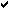# * Astronomy

 Home -> Astronomy News -> Stars/galaxy News -> PSR B1516+02B
Post Info TOPIC: PSR B1516+02BLPosts: 131433
Date:
 PSR B1516+02B Permalink Title: Super-Massive Neutron StarsAuthors: Paulo C. C. FreireWe present here the results of Arecibo timing of PSR B1516+02B, a 7.95-ms pulsar in a binary system with a ~0.17 solar mass companion and an orbital period of 6.85 days located in the globular cluster M5. The eccentricity of the orbit (e = 0.14) has allowed a measurement of the rate of advance of periastron: (0.0136 ± 0.0007) degrees per year. It is very likely that the periastron advance is due to the effects of general relativity; the total mass of the binary system is (2.14 ±0.16) solar masses. The small measured mass function implies, in a statistical sense, that a very large fraction of this total mass is contained in the pulsar: (1.94+0.17 -0.19) solar masses (1-sigma); there is a 5% probability that the mass of this object is below 1.59 solar masses. With the possible exception of PSR J1748-2021B, this is the largest neutron star mass measured to date. When combined with similar measurements made previously for Terzan 5 I and J, we can exclude, in a statistical sense, the "soft" equations of state for dense neutron matter, implying that matter at the centre of a neutron star is highly incompressible. There is also some evidence for a bimodal distribution of MSP masses, the reasons for that are not clear. Read more (76kb, PDF) __________________
 Page 1 of 1  sorted by Oldest FirstNewest First